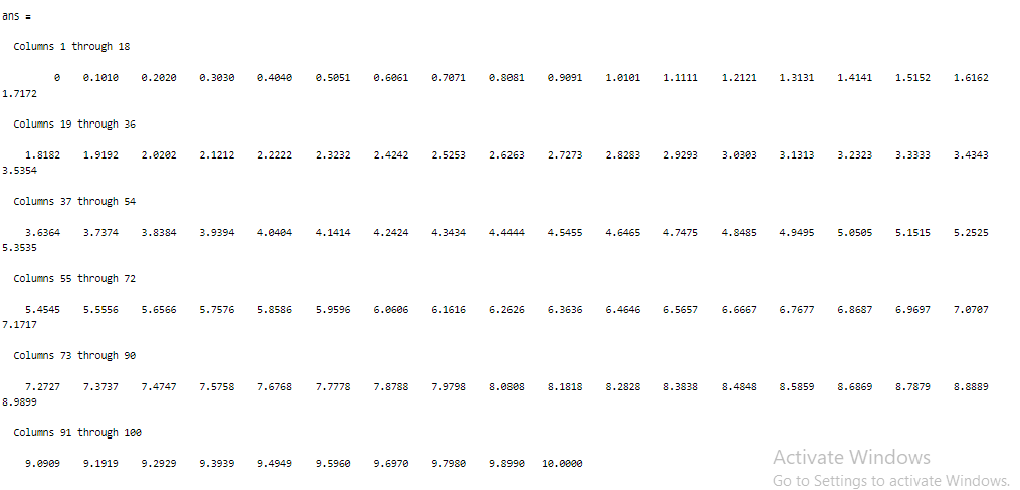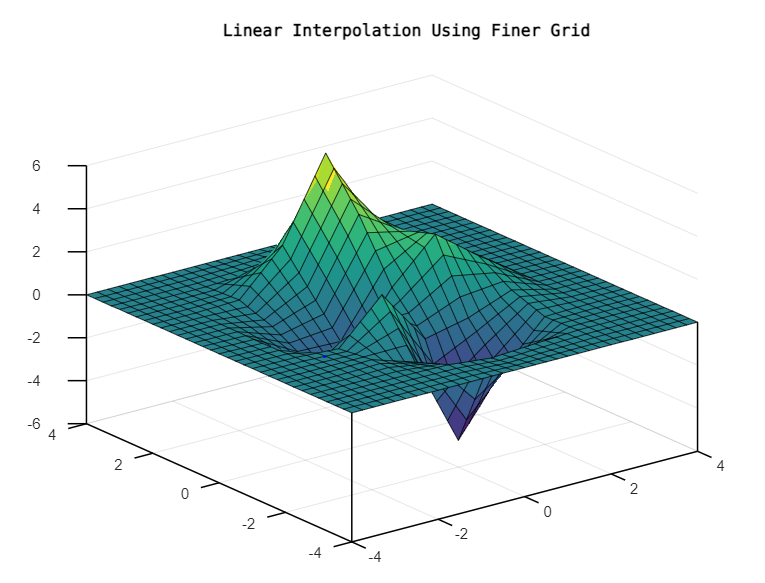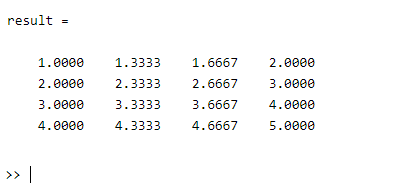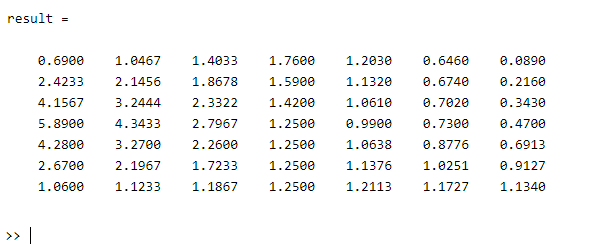Open in App
Not now

# 2D Array Interpolation in MATLAB

• Last Updated : 06 Dec, 2022

In this article, we are going to discuss “2D Array Interpolation” in MATLAB with the help of two linspace() and interp2() functions.

## Functions Used

### linspace( )

The linspace() function is used for the generation linearly spaced vector.

Syntax:

linspace(a, b)

linspace(a, b, n)

Here,

linspace(a, b) is used to return a row vector of 100 evenly spaced points in between “a” and “b”.

linspace(a, b, n) is used to return a row vector of “n” points, the spacing between the points is (x2-x1)/(n-1).

Parameters: This function accepts three parameters, which are illustrated below:

• a: This is the specified first value.
• b: This is the specified second value.
• n: This is the specified number of points contained in the generated row vector.

Return Value: It returns the generated row vector of “n” points linearly spaced between and including “a” and “b”.

Example:

## Matlab

 `% MATLAB code for Calling the linspace() ` `% to generate a row vector of ` `% 100 evenly spaced points in` `% between 0 to 10` `linspace(0, 10)`

Output:### interp2( )

The interp2() function is used to Interpolate for 2-D gridded data in a mesh grid format.

Syntax:

interp2(X,Y,V,Xq,Yq)

interp2(V,Xq,Yq)

interp2(V)

interp2(V,k)

interp2(___,method)

interp2(___,method,extrapval)

Here,

interp2(X, Y, V, Xq, Yq) function is used to return interpolated values of a specified function of two variables at specific query points using linear interpolation. Its result passes through the original sampling of the function. Here the coordinates of the sample points reside in “X” and “Y”, “V” contains the corresponding function values at each sample point, and “Xq”, “Yq” contains the coordinates of the query points.

interp2(V, Xq, Yq) function is for the default grid of sample points. These points cover the rectangular region, X=1:n and Y=1:m, where [m,n] = size(V).

interp2(V) function is used to return the interpolated values on a refined grid formed by dividing the interval between sample values once in each dimension.

interp2(V, k) function is used to return the interpolated values on a refined grid formed by repeatedly halving the intervals k times in each dimension. This results in 2^k-1 interpolated points between sample values.

interp2(___, method) function specifies an alternative interpolation function such as ‘linear’, ‘nearest’, ‘cubic’, ‘makima’, or ‘spline’. Its default value is ‘linear’.

interp2(___, method, extrapval) function specifies ‘extrapval ‘, which is a scalar value assigned to all queries that lie outside the domain of the sample points.

Parameters: This function accepts some parameters which are illustrated below:

• X: It is the first coordinate for the sample point.
• Y: It is the second coordinate for the sample point.
• V: This is used as size(V) which is equivalent to [m, n].
• Xq: This contains the coordinates of the query points which is equivalent to X=1:n
• Yq: This contains the coordinates of the query points which is equivalent to X=1:m
• k: It is the interval.
• method: It specifies an alternative interpolation function such as ‘linear’, ‘nearest’, ‘cubic’, ‘makima’, or ‘spline’. Its default value is ‘linear’.
• extrapval: It is a scalar value assigned to all queries that lie outside the domain of the sample points.

Return Value: It returns the interpolated 2-D array.

Example:

## Matlab

 `% MATLAB code for interp2()` `% Specifying a 2-D grid` `[X,Y] = meshgrid(-4:4);`   `% Sampling the peaks() function` `V = peaks(X,Y);` `[Xq,Yq] = meshgrid(-4:0.25:4);`   `% Calling the interp2() function` `title(``'Linear Interpolation Using Finer Grid'``);` `Vq = interp2(X,Y,V,Xq,Yq);`

Output:Example 1:

## Matlab

 `% MATLAB code for linspace() to generate` `% row vectors of 4 points` `% Initializing a 2d array of dimensions 3*3` `A=[1 2 3` `   ``4 5 6` `   ``7 8 9];` `   `  `% Calling the linspace() function to` `% generate the row vectors of 4 points` `% in between and including 1 to 2` `x = linspace(1, 2, 4); `   `% Calling the interp2() function for` `% interpolation of above specified 2d array ` `result = interp2(A, x(:), x)`

Output:Example 2:

## Matlab

 `% MATLAB code for linspace() and interp2() ` `% Initializing a 2d array of dimensions 4*4` `A=[0.69    1.76    0.089    2.0012;` `   ``5.89    1.25    0.47     0.55;` `   ``1.06    1.25    1.134    4.163;` `   ``2       1.7     0        2.4];` `   `  `% Calling the linspace() function to` `% generate the row vectors of 7 points` `% in between and including 1 to 3` `x = linspace(1, 3, 7); `   `% Calling the interp2() function for` `% interpolation of above specified 2d array ` `result = interp2(A, x(:), x)`

Output:My Personal Notes arrow_drop_up
Related Articles